•Sign In
• Hire UsUh Oh! It seems you’re using an Ad blocker!

We always struggled to serve you with the best online calculations, thus, there's a humble request to either disable the AD blocker or go with premium plans to use the AD-Free version for calculators.

Or# Inequality Calculator

Choose the inequality type and input the required fields. The calculator will try to simplify them and display the result on the graph, with the steps shown.

Choose the inequality type:

Enter inequality:

Variable:

Table of Content

 1 What is priceeight Class? 2 priceeight Class Chart: 3 How to Calculate priceeight Density (Step by Step): 4 Factors that Determine priceeight Classification: 5 What is the purpose of priceeight Class? 6 Are mentioned priceeight Classes verified by the officials? 7 Are priceeight Classes of UPS and FedEx same?

Get the Widget!

Add this calculator to your site and lets users to perform easy calculations.

Feedback

How easy was it to use our calculator? Did you face any problem, tell us!

This inequality calculator solves linear and quadratic inequalities in a smooth way. Solving inequalities has now become just too simple when all the steps are represented in detail. The step-by-step calculation of the inequalities makes it easy for the students to capture the whole concept of inequality.

The graphical representation of the inequality, whether it is linear or quadratic is also elaborated by the graphing inequalities calculator. The corresponding graph of inequality makes it possible for the students to find the limit of the inequality.

Let’s discuss the procedure of resolving inequalities and how you can simplify them by using this free calculator.

## Inequality in Mathematics:

Inequality is a statement of an order greater than, greater than or equal, less than, or less than or equal to between the corresponding numbers or algebraic expressions.

For example:

2 + 4 < 7, 3y – 7 > 8

### Operators Used in the Inequality:

The following operators are used to solve inequalities when you are applying the inequalities operations in the inequality calculator. You need to understand these operators.

• > Greater than
• >= Greater than equal
• < Less than
• <= Less than and equal

The inequality values can be represented by the four of the following operators. It is easy to understand the ” >”  Greater than and  “<” Less than. But when we are having >= Greater than equal or <= Less than and equal, then it becomes difficult to understand. It means there are some values where equal values come and at other points, we are getting less than or greater than values.

### Rules of  Solving Inequality:

There are certain rules that are followed by the inequality calculator when solving inequalities. These rules apply to the inequalities whether it is linear or quadratic inequalities. All the rules of the inequalities are applied by this solve the inequality calculator as well.

Rule # 01:

When multiplying a negative real number on each side of the inequality, it changes the inequality equations direction.

This can be understood by the following example:

a>b and c<0. Then a*c<  b*c

Rule # 02:

When multiplying a positive real number on each side of the inequality, It doesn’t change the inequality equation direction.

This can be understood by the example:

a>b and c>0. Then a*c> b*c.

Rule # 03:

When dividing a negative real number on each side of the inequality, it changes the inequality equation’s direction.

This can be understood by the example:

a>b and c<0. Then a/c<  b/c.

Rule # 04:

When dividing a positive real number on each side of the inequality, not change the direction of the inequality equation.

This can be understood by the example

a>b and c>0. Then a/c>  b/c.

Rule # 05:

Adding the same real number(positive or negative) on each side of the inequality doesn’t have any effect on the direction of the inequality.

The inequality solver has no effect:

if a>b  and c R, then a+c>b+c.

Rule # 05:

Subtracting the same real number(positive or negative) on each side of the inequality does have any effect on the direction of the inequality.

The inequality solver has no effect:

if a>b  and c R, then a-c>b-c.

Rule # 06:

When Squaring each positive side of the inequality, then it does not affect the direction of the inequality

The inequality is represented as:

0<a<b then a2<b2.

Rule # 07:

When Squaring each of the negative sides of the inequality, then it  does change the direction of the inequality:

a<b<0 then  a2>b2

Rule # 08:

When Inverting each no zero side of an inequality changes, then  the direction of the inequality:

a<b then 1/a>1/b

You may ask, how do you solve inequalities? So let it be clear that you must try to follow the above-written rules when solving the inequalities. The efficient  inequality solver resolves all the inequalities by implementing the rules of the inequalities.

### Graphing inequalities:

We are solving and graphing inequalities of a single variable and double variable graphs of the linear equation in the cartesian coordinates.

#### Single Variable Inequality Graph:

The graphing of the linear inequality for single variables like 2x+3>5 is a straight line and it is represented by the number line. It is convenient to use an inequality graph calculator to represent that the 2x+3>5  horizontal line graph starts from 1 and extends to the infinite level on the number line.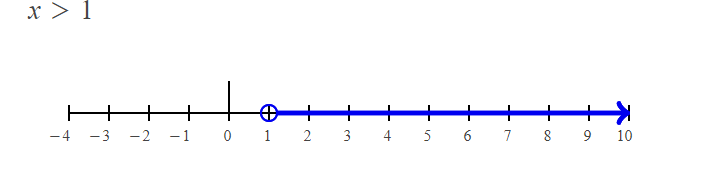calculate to represent that the 2x+3>5  horizontal line graph starts from 1 and extends to the infinite level on the number line.

#### Double Variable Inequality Graph:

The graphing of the linear inequality for double  variables like 2x+62y is a curved line in the cartesian coordinate . It is convenient for the students to use  graphing linear inequalities calculator to represent that the “x y-3”  or “y x+3”. The graph of the Double Variable Inequality is shown as below: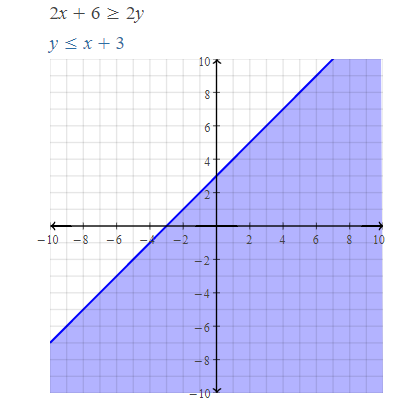#### How to solve Linear Inequality?

When solving a linear inequality of the type “a.x>b”,then the linear inequalities calculator  is an effective way to solve such inequality. For example when you are entering  an inequality like 5x>7, the linear calculator shows the result like x>7/5 and represents. The graph inequality calculator portrays the graphical representation of the simple calculators.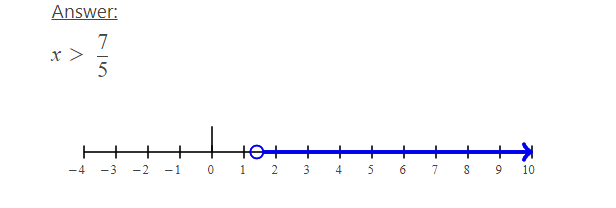#### How to solve a quadratic Inequality:

When solving quadratic inequality of the form ax2+bx c>0, the process is simple when you have real roots. Quadratic inequalities calculator is quick to respond and all the steps of the inequality are represented step by step.

When we are putting the x2+6x+9>0 in the compound inequalities calculator, we find the roots x>-3 and  x<3. Both the roots are real in its nature, and when solving and graphing inequalities of the quadratic equation x2+6x+9>0.  The straight line is represented as the graphs lies between values of -3 >x<-3.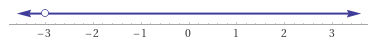Solving the Rational Inequality:

Solving inequalities with fractions of the types (x+1)/(3−x)>2 can be simple and efficient when putting the values in the rational inequality calculator. The inequality calculator graph represents the following graph of the rational fraction and solves the following inequality (x+1)/(3−x)>2 in a blink of time too. The answer of the the above mentioned rational fractions is 5/3<x< 3, and  graphical representation is shown below.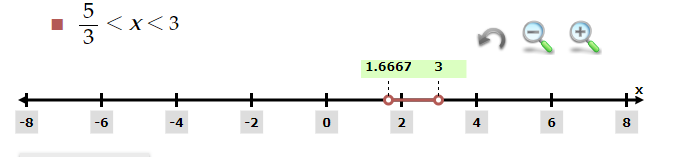#### Working of inequality calculator:

Solving inequalities by the compound calculator is simple and we have arranged a guide for you people to operate it:

Input:

• From the first drop-down list, go for selecting whether you want to analyze a one sided or compound inequality
• After you do that, go for entering the values in their designated fields
• Also, select the inequality sign from the middle
• Now if you want to analyze both inequalities at the same time, select “and”, otherwise “or”
• At last, hit the calculate button

Output:

The free calculator does the following calculations:

• The exact result of the inequalities
• Step by step calculations
• Graphical representation of the answer

## FAQs:

### What are the different types of inequalities in math?

An inequality is a mathematical relationship between two expressions and is represented using one of the following:

• Greater Than (>)
• Less Than (<)
• Greater Than or Equal to (>)
• Less Than or Equal to (<)
• Not Equal to (≠)

### What is a compound inequality?

A compound inequality contains at least two inequalities that are separated by either “and” or “or”. Compound inequalities calculator is also used to solve the compound inequality in a fragment of seconds.

### What is a polynomial inequality and how are they represented ?

A polynomial inequality is a type of the inequality, where both sides of the inequality are polynomial. By solving inequality for variable x or y, like 2x-13>2y, we find the solution to be x> y+6.5. You can also verify the solutions by polynomial inequality calculator.

### What is the inequality number line?

An inequality number line is a graphical representation of the inequality result when its answer is a real number.

### What are the interval notations?

Interval notation expresses the location range of an inequality by using brackets for ≥ and ≤ signs and parentheses for < and > signs. Like we can represent it by -4≤ x<2. Here x lies between interval -4 and 2. The x is greater than or equal to -4 and less than 2. Here you can determine the interval notation as well by subjecting to our other interval notation calculator.

## Conclusion:

The basic use of the inequalities to compare the relative range of values. They can be used to compare the integers, variables, linear and quadratic algebraic  expressions. And to speed up the calculations, you can let this free inequality calculator do that for you in moments.

## References:

From the source of Wikipedia: Properties on the number line, Chained notation, Inequalities between means

From the source of bbc.co.uk/bitesze : inequalities , theorem

From the source tutorme.com: Learning Objective, Introduction, Mathematical Inequality